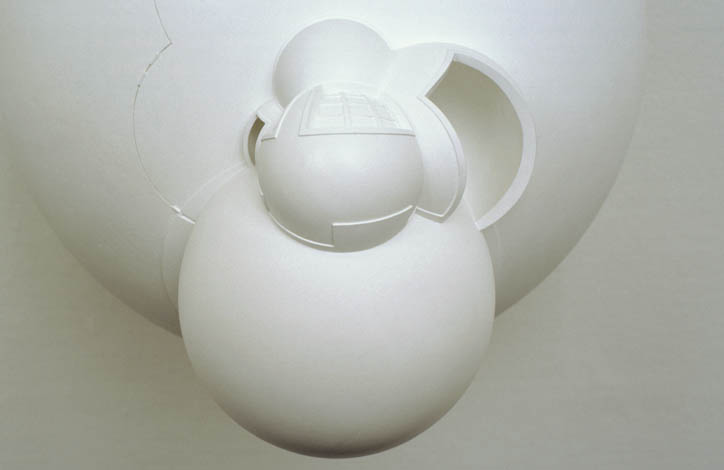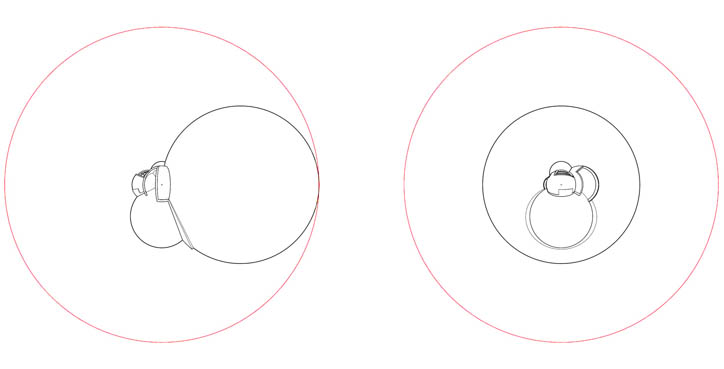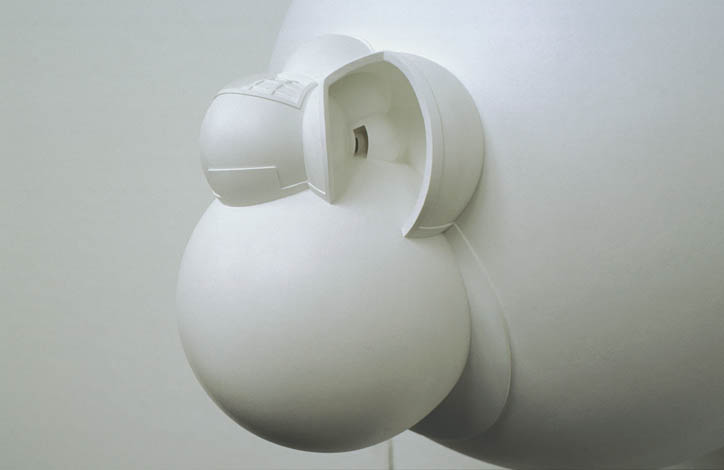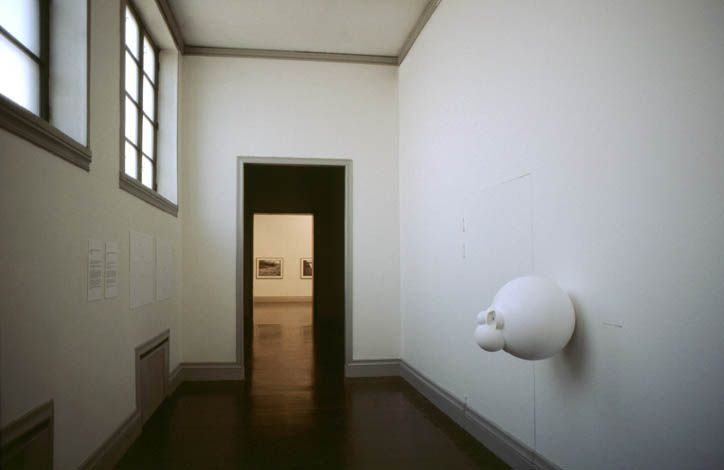# Prototype N60°10’19.0323”; E24°56’4.2900”; +21.84m###### 1998

In this work, the absolute center point of the universe is determined as N60°10´19.0323´´; E24°56´4.2900´´; +21.84m, and the principle is to present how the world appears when observed from this point. In the work the universe is turned inside out relative to a unit ball(*) (diameter 1 metre) according to the following formula:
$P(\vec{x}) = \frac{\vec{x}·x_0^2}{|x|^2}$ where $x_0$ (value 0.5m) is the radius of the unit ball, and $\vec{x}$ is a vector from the center point of the unit ball, which is N60°10´19.0323´´; E24°56´4.2900´´; +21.84m. This means that the space outside the unit ball is transferred in its entirety into the inner space of the ball and vice versa (the infinity of space becomes the center point and the center point infinity, while points on the surface of the unit ball remain where they are). Thus placed in its true position the three-dimensional “map of the universe” becomes “identical” with the real world through this formula.

In this representation, as also in central perspective in general, the dimensions of space change in inverse relation to the distance defined from the center or perception point. Thus, in principle, the work can be thought of being a “precise” central perspective map of the universe (when observing the universe from this point).

######(*) To illustrate this matter the unit ball (diameter 1 meter) has been marked as red circles in the drawings illustrating the room (above) and its projection (below). The drawings are to different scales.